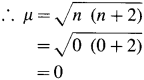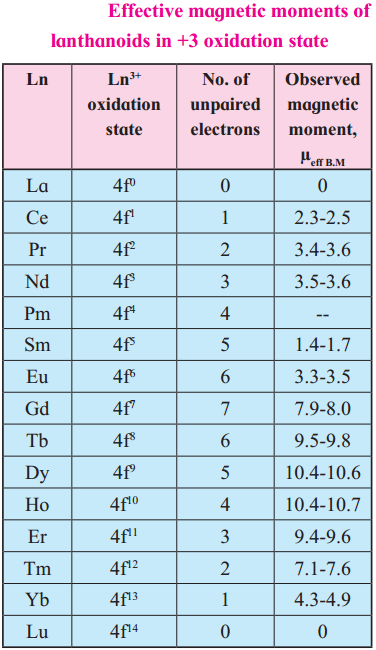# Maharashtra Board Class 12 Chemistry Solutions Chapter 8 Transition and Inner Transition Elements

Balbharti Maharashtra State Board 12th Chemistry Textbook Solutions Chapter 8 Transition and Inner Transition Elements Textbook Exercise Questions and Answers.

## Maharashtra State Board Class 12 Chemistry Solutions Chapter 8 Transition and Inner Transition Elements

1. Choose the most correct option.

Question i.
Which one of the following is diamagnetic
a. Cr3⊕
b. Fe3⊕
c. Cu2⊕
d. Sc3⊕
d. Sc3⊕Question ii.
Most stable oxidation state of Titanium is
a. +2
b. +3
c. +4
d. +5
c. +4

Question iii.
Components of Nichrome alloy are
a. Ni, Cr, Fe
b. Ni, Cr, Fe, C
c. Ni, Cr
d. Cu, Fe
(c) Ni, Cr

Question iv.
Most stable oxidation state of Ruthenium is
a. +2
b. +4
c. +8
d. +6
(b) +4

Question v.
Stable oxidation states for chromium are
a. +2, +3
b. +3, +4
c. +4, +5
d. +3, +6
d. +3, +6

Question vi.
Electronic configuration of Cu and Cu+1
a. 3d10, 4s0; 3d9, 4s0
b. 3d9, 4s1; 3d94s0
c. 3d10, 4s1; 3d10, 4s0
d. 3d8, 4s1; 3d10, 4s0
c. 3d10, 4s1; 3d10, 4s°

Question vii.
Which of the following have d0s0 configuration
a. Sc3⊕
b. Ti4⊕
c. V5⊕
d. all of the above
d. All of the above

Question viii.
Magnetic moment of a metal complex is 5.9 B.M. Number of unpaired electrons in the complex is
a. 2
b. 3
c. 4
d. 5
d. 5

Question ix.
In which of the following series all the elements are radioactive in nature
a. Lanthanoids
b. Actinoids
c. d-block elements
d. s-block elements
b. Actinides

Question x.
Which of the following sets of ions contain only paramagnetic ions
a. Sm3⊕, Ho3⊕, Lu3⊕
b. La3⊕, Ce3⊕, Sm3⊕
c. La3⊕, Eu3⊕, Gd3⊕
d. Ce3⊕, Eu3⊕, Yb3⊕
d. Ce3⊕, Eu3⊕, Yb3⊕Question xi.
Which actinoid, other than uranium, occur in a significant amount naturally?
a. Thorium
b. Actinium
c. Protactinium
d. Plutonium
a. Thorium

Question xii.
The flux added during extraction of Iron from hematite are its?
a. Silica
b. Calcium carbonate
c. Sodium carbonate
d. Alumina
b. Calcium carbonate

Question i.
What is the oxidation state of Manganese in
$$\text { (i) } \mathrm{MnO}_{4}^{2-}(\mathrm{ii}) \mathrm{MnO}_{4}^{-} \text {? }$$
Oxidation state of Manganese in
$$(i) \mathrm{MnO}_{4}^{2-} is +6 (ii) \mathrm{MnO}_{4}^{-}is +7$$

*Question ii.
Give uses of KMnO4

Question iii.
Why salts of Sc3⊕, Ti4⊕, V5⊕ are colorless?
(i) Sc3+ salts are colourless :

• The electronic configuration of 21Sc [Ar| 3d1 4s2 and Sc3+ [Ar] d°.
• Since there are no unpaired electrons in 3d subshell, d → d transition is not possible.
• Therefore, Sc3+ ions do not absorb the radiations in the visible region. Hence salts of Sc3+ are colourless (or white).

(ii) Ti4+ salts are colourless :

• The electronic configuration of 22Ti [Ar] 3d24s2 and Ti4+ : [Ar] d°
• Since there are no unpaired electrons in 3d subshell, d-*d transition is not possible.
• Therefore, Ti3+ ions do not absorb the radiation in visible region. Hence salts of Ti3+ are colourless.

(iii) Vs5+ salts are eolourless :

• The electronic configuration of 23V : [Ar] 3d34s2 and V5+ : [Ar] 3d°
• Since there are no unpaired electrons in 3d-subshell, d – d transition is not possible.
• Therefore, V5+ ions do not absorb the radiations in the visible region. Hence, V5+ salts are colourless, a

Question iv.
Which steps are involved in the manufacture of potassium dichromate from chromite ore?
Steps in the manufacture of potassium dichromate from chromite ore are :

• Concentration of chromite ore.
• Conversion of chromite ore into sodium chromate (Na2CrO4).
• Conversion of Na2CrO4 into sodium dichromate (Na2Cr2O7).
• Conversion of Na2Cr2O7 into K2Cr2O7.

Question v.
Balance the following equation
(i) KMnO4 + H2C2O4 + H2SO4 → MnSO4 + K2SO4 + H2O + O2
(ii) K2Cr2O7 + KI + H2SO4 → K2SO4 + Cr2(SO4)3 + 7H2O + 3I2
(i) 2KMnO4 + 3H2SO4 + 5H2C2O4 → K2SO4 + 2MnSO4 + 8H2O + 10CO2
(ii) Acidified potassium dichromate oxidises potassium iodide (KI) to iodine (I2). Potassium dichromate is reduced to chromic sulphate. Liberated iodine turns the solution brown K2Cr2O7 + 6KI + 7H2SO4 → 4K2SO4 + Cr2(SO4)3 + 7H2O + 3I2 [Oxidation state of iodine increases from – 1 to zero]Question vi.
What are the stable oxidation states of plutonium, cerium, manganese, Europium?
Stable oxidation states :
Plutonium + 3 to + 7
Cerium + 3, + 4
Manganese + 2, + 4, + 6, + 7
Europium +2, +3

Question vii.
Write the electronic configuration of chromium and copper.
Chromium (24Cr) has electronic configuration,
24Cr (Expected) : Is2 2s2 2p6 3s2 3p6 3d4 4s2
(Observed) : Is2 2s2 2p6 3s2 3p6 3d5 4s1

Explanation :

• The energy difference between 3d- and 45-orbitals is very low.
• d-orbitals being degenerate, they acquire more stability when they are half-filled (3d5).
• Therefore, there arises a transfer of one electron from 45-orbital to 3d-orbital in Cr giving more stable half-filled orbital. Hence, the configuration of Cr is [Ar] 3d5 4s1 and not [Ar] 3d4 4s2.

Copper (29CU) has electronic configuration,
29Cu (Expected) : Is2 2s3 2p6 3s3 3p6 3d9 4s2
(Observed) : Is2 2s2 2p6 3s2 3p6 3d10 4s1

Explanation :

• The energy difference between 3d- and 45-orbitals is very low.
• d-orbitals being degenerate, they acquire more stability when they are completely filled.
• Therefore, there arises a transfer of one electron from 45-orbital to 3d-orbital in Cu giving completely filled more stable d-orbital.

Hence, the configuration of Cu is [Ar] 3d10 4s1 and not [Ar] 3d9 4s2.

Question viii.
Why nobelium is the only actinoid with +2 oxidation state?

• Nobelium has the electronic configuration 102NO : [Rn] 5f146d°7s2
• No2+ : [Rn] 5f146d°
• Since the 4f subshell is completely filled and 6d° empty, + 2 oxidation state is the stable oxidation state.
• Other actinoids in + 2 oxidation state are not as stable due to incomplete 4f subshell.

Question ix.
Explain with the help of balanced chemical equation, why the solution of Ce(IV) is prepared in acidic medium.
Ce4+ undergoes hydrolysis as, Ce4++ 2H2O → Ce(OH)4 + 4H+.
Due to the presence of H+ in the solution, the solution is acidic.

Question x.
What is meant by ‘shielding of electrons’ in an atom?
The inner shell electrons in an atom screen or shield the outermost electron from the nuclear attraction. This effect is called the shielding effect.

The magnitude of the shielding effect depends upon the number of inner electrons.

Question xi.
The atomic number of an element is 90. Is this element diamagnetic or paramagnetic?
The 90th element thorium has an electronic configuration, [Rn] 6d27s2. Since it has 2 unpaired electrons it is paramagnetic.

Question i.
Explain the trends in atomic radii of d-block elements

1. The atomic or ionic radii of 3-d series transition elements are smaller than those of representative elements, with the same oxidation states.
2. For the same oxidation state, there is an increase in nuclear charge and a gradual decrease in ionic radii of 3d-series elements is observed. Thus ionic radii of ions with oxidation state + 2 decreases with increase in atomic number.
3. There is slight increase is observed in Zn2+ ions. With the higher oxidation states, effective nuclear charge increases. Therefore ionic radii decrease with increase in oxidation state of the same element. For example, Fe2+ ion has ionic radius 77 pm whereas Fe3+ has 65 pm.Question ii.
Name different zones in the Blast furnace. Write the reactions taking place in them.
(i) Zone of combustion : The hot air oxidises coke to CO which is an exothermic reaction, due to which the temperature of furnace rises.
C + 1/2 O2 → CO ΔH= – 220kJ
Some part of CO dissociates to give finely divided carbon and O2.
2CO → 2C + O2
The hot gases with CO rise up in the furnace and heats the charge coming down. CO acts as a fuel as well as a reducing agent.

(ii) Zone of reduction : At about 900 °C, CO reduces Fe2O3 to spongy (or porous) iron.
Fe2O3 + 3CO → 2Fe + 3CO2
Carbon also reduces partially Fe203 to Fe.
Fe2O3 + 3C → 2Fe + 3CO

(iii) Zone of slag formation : At 1200 K limestone, CaCO3 in the charge, decomposes and forms a basic flux CaO which further reacts at 1500 K with gangue (SiO2, Al2O3) and forms a slag of CaSiO3 and Ca3AlO3.
CaCO3 + CaO + CO2.
CaO + SiO2 → CaSiO3
12CaO + 2Al2O3 → 4Ca3AlO3 + 3O2

The slag is removed from the bottom of the furnace through an outlet.

(iv) Zone of fusion : The impurities in ore like MnO2 and Ca3(PO4)2 are reduced to Mn and P while SiO2 is reduced in Si. The spongy iron moving down in the furnace melts in the fusion zone and dissolves the impurities like C, Si, Mn, phosphorus and sulphure. The molten iron collects at the bottom of furnace. The lighter slag floats on the molten iron and prevents its oxidation.

The molten iron is removed and cooled in moulds. It is called pig iron or cast iron. It contains about 4% carbon.

Question iii.
What are the differences between cast iron, wrought iron and steel?

 Cast iron Wrought iron Steel (1) Hard and brittle (2) Contains 4% carbon. (3) Used for making pipes, manu­facturing automotive parts, pots, pans, utensils (1) Very soft (2) Contains less than 0.2% carbon. (3) Used for making pipes, bars for stay bolts, engine bolts and rivets. (1) Neither too hard nor too soft. (2) Contains 0.2 to 2% carbon (3) Used in buildings infrastruc­ture, tools, ships, automobiles, weapons etc.

Question iv.
Iron exhibits +2 and +3 oxidation states. Write their electronic configuration. Which will be more stable? Why?
The electronic configuration of Fe2 + and Fe3+ :
Fe2+ : Is2 2s2 2p6 3s2 3p6 3d6
Fe3+ : Is2 2s2 2p6 3s2 3p6 3d5

Due to loss of two electrons from the 4.v-orbital and one electron from the 3d-orbital, iron attains 3+ oxidation state. Since in Fe3+, the 3d-orbital is half-filled, it gets extra stability, hence Fe3+ is more stable than Fe2+.

Question v.
Give the similarities and differences in elements of 3d, 4d and 5d series.
Similarity :

• They are placed between .s-block and p-block of the periodic table.
• All elements are metals showing metallic characters.
• Some are paramagnetic.
• Most of them give coloured compounds.
• They have catalytic properties.
• They form complexes.
• They have variable oxidation states.

Differences :

• In 4d and 5d series lanthanide and actinoid contraction is observed. In 3d series atomic size changes are less marked.
• 4d and 5d elements have high coordination numbers compared to 3d elements.
• 4d and 5d series have similar properties whereas 3d series have different properties.

Question vi.
Explain trends in ionisation enthalpies of d-block elements.

1. The ionisation enthalpies of transition elements are quite high and lie between those of 5-block and p-block elements. This is because the nuclear charge and atomic radii of transition elements lie between those of 5-block and p-block elements.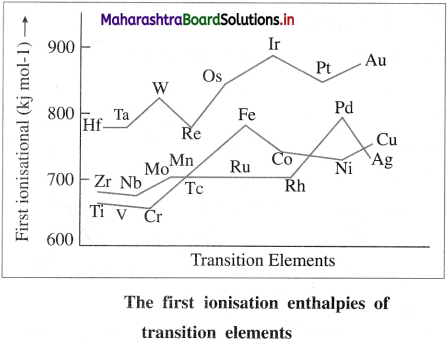2. As atomic number of transition elements increases along the period and along the group, first ionisation enthalpy increases even though the increase is not regular.
3. If IE1; IE2 and IE3 are the first, second and third ionisation enthalpies of the transition elements, then IE1 < IE2 < IE3.
4. In the transition elements, the added last differentiating electron enters into (n – 1) d-orbital and shields the valence electrons from the nuclear attraction. This gives rise to the screening effect of (n – 1) d-electrons.
5. Due to this screening effect of (n – 1) d electrons, the ionisation enthalpy increases slowly and the increase is not very regular.Question vii.
What is meant by diamagnetic and paramagnetic metal? Give one example of diamagnetic and paramagnetic transition metal and lanthanoid metal.

1. Paramagnetic substances : When a magnetic field is applied, substances which are attracted towards the applied magnetic field are called paramagnetic substances. Example : Ni2+, Pr4+
2. Diamagnetic substances : When a magnetic field is applied, substances which are repelled by the magnetic fields are called diamagnetic substances. Example : Zn2+, La3+
3. Ferromagnetic substances : When a magnetic field is applied, substances which are attracted very strongly are called ferromagnetic substances. These substances can be magnetised. For example, Fe, Co, Ni are ferromagnetic.

Question viii.
Why the ground-state electronic configurations of gadolinium and lawrencium are different than expected?

Question ix.
Write steps involved in the metallurgical process
The various steps and principles involved in the extraction of pure metals from their ores are as follows.:

• Concentration of ores in which impurities (gangue) are removed.
• Conversion of ores into oxides or other reducible compounds of metals.
• Reduction of ores to obtain crude metals.
• Refining of metals giving pure metals.

Question x.
Cerium and Terbium behaves as good oxidising agents in +4 oxidation state. Explain.

• The most stable oxidation state of lanthanoids is +3.
• Hence, Ce4+ (cerium) and Tb4+ (terbium) tend to get + 3 oxidation state which is more stable.
• Since they get reduced by accepting electron, they are good oxidising agents in + 4 oxidation state.

Question xi.
Europium and Ytterbium behave as good reducing agents in +2 oxidation state explain.

• The most stable oxidation state of lanthanoids is + 3.
• Hence, Eu2+ and Yb2+ tend to get + 3 oxidation states by losing one electron.
• Since they get oxidised, they are good reducing agents in + 2 oxidation state.

Activity :
Make groups and each group prepare a PowerPoint presentation on the properties and applications of one element. You can use your imagination to create some innovative ways of presenting data.

You can use pictures, images, flow charts, etc. to make the presentation easier to understand. Don’t forget to cite the reference(s) from where data for the presentation is collected (including figures and charts). Have fun!

12th Chemistry Digest Chapter 8 Transition and Inner Transition Elements Intext Questions and Answers

Do you know? (Textbook Page No 165)

Question 1.
In which block of the modern periodic table are the transition and inner transition elements placed?
The transition elements are placed in d-block and inner transition elements are placed in f-block of the modern periodic table.

Use your brain power! (Textbook Page No 167)

Question 1.
Fill in the blanks with correct outer electronic configurations.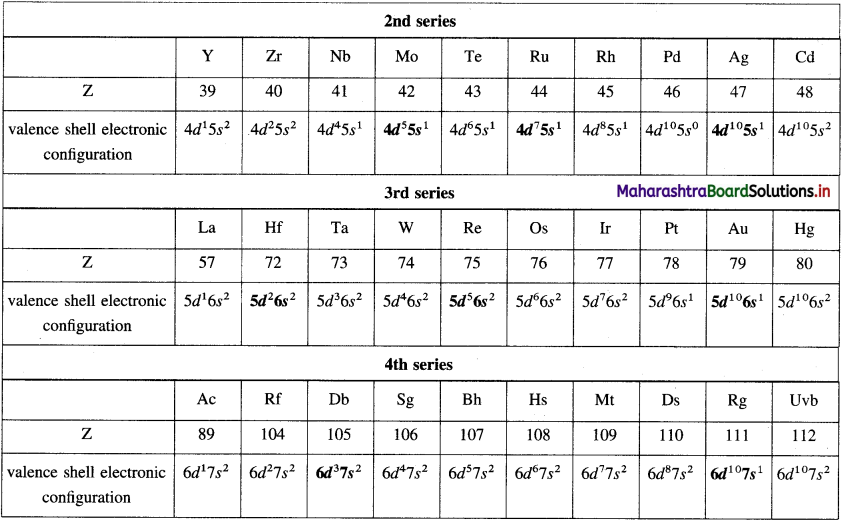Try this….. (Textbook Page No 168)

Question 1.
Write the electronic configuration of Cr and Cu.
24Cr : [Ar] 3d54s1 30Cu : [Ar] 3d104s1

Can you tell? (Textbook Page No 168)

Question 1.
Which of the first transition series element shows the maximum number of oxidation states and why?

• 25Mn shows the maximum number of oxidation states, + 2 to +7.
• 25Mn : [Ar] 3d54s3
• Mn has incompletely filled J-subshell.
• Due to small difference in energy between 3d and 4s -orbitals, Mn can lose (or share) electrons from both the orbitals.
• Hence Mn shows oxidation states from + 2 to +7.

Question 2.
Which elements in the 4d and 5d-series will show maximum number of oxidation states?
In 4d-series maximum number of oxidation states are for Ruthenium Ru ( + 2, +3, + 4„ +6, +7, + 8). In 5d-series, maximum number of oxidation states are for Osmium, Os ( + 2 to + 8).

Try this ….. (Textbook Page No 168)

Question 1.
Write the electronic configuration of Mn6+, Mn4+, Fe4+, Co5+, Ni2+.

 Ions Electronic configuration of valence shell Mn6+ [Ar] 3d1 Mn4+ [Ar] 3d3 Fe4+ [Ar] 3d4 Co5+ [Ar] 3d4 Ni2+ [Ar] 3d8

Try this ….. (Textbook Page No 171)

Question 1.
Pick up the paramagnetic species from the following : Cu1+, Fe3+, Ni2+, Zn2+, Cd2+, Pd2+.
The following ions are paramagnetic : Fe3+, Ni2+, Pd2+

Try this ….. (Textbook Page No 171)

Question 1.
What will be the magnetic moment of transition metal having 3 unpaired electrons?
(a) equal to 1.73 B.M.?
(b) less than 1.73 BM.
(c) more than 1.73 B.M.?
By spin-only formula, $$\mu=\sqrt{n(n+2)}$$ where n is number of unpaired electrons.
$$\mu=\sqrt{3(3+2)}=\sqrt{3(5)}=3.87 \mathrm{~B} . \mathrm{M}$$
Thus the value is more than 1.73 B.M.

Use your brain power! (Textbook Page No 171)

Question 1.
A metal ion from the first transition series has two unpaired electrons. Calculate the magnetic moment.
\begin{aligned}
\mu &=\sqrt{n(n+2)} \\
&=\sqrt{2(2+2)} \\
&=\sqrt{8} \\
&=2.84 \text { B.M. }
\end{aligned}Problem (Textbook Page No 172)

Question 1.
Calculate the spin-only magnetic moment of divalent cation of a transition metal with atomic number 25.
For element with atomic number 25. electronic configuration of its divalent cation will be : [Ar] 3d5.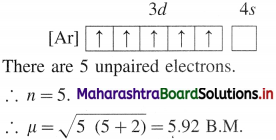Try this….. (Textbook Page No 172)

Question 1.
Calculate the spin-only magnetic moment of a divalent cation of element Slaving atomic number 27.
Electronic configuration of divalent ion of an element with atomic number 27 : [Ar] 3d7;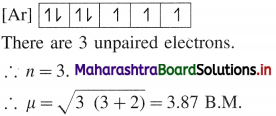Can you tell? (Textbook Page No 172)

Question 1.
Compounds of s and p-block elements are almost white. What could be the absorbed radiation? (uv or visible)?
The white colour of a compound indicates the absorption of uv radiation.

Can you tell? (Textbook Page No 181)

Question 1.
Why f-block elements are called inner transition metals?
f-block elements are called inner transition elements since f-orbital lies much inside the f-orbital in relation to the transition metals, These elements have 1 to 14 electrons in their f-orbital.

Question 2.
Are there an similarities between transition and inner transition metals?
There are some properties similarity between transition and inner transition metals.

• They are placed between s and p-block elements.
• They are metals with filling of inner suhshells in their electronic configuration.
• They show variable oxidation slates.
•  They show magnetism.
• They form coloured compounds.
• They have catalytic property.

Problem (Textbook Page No 184)

Question 1.
Which of the following will have highest fourth ionisation enthalpy, La4+, Gd4+, Lu4+.
La : 4f°5d16s2
Gd : 4f15d16s2
Lu : 4f145d16s2
Lu will have the highest fourth ionisation enthalpy since Lu3+ has the most stable configuration of 4f14.

Use your brain power! (Textbook Page No 185)

Question 1.
Do you think that lanthanoid complex would show magnetism?
Lanthanoid complexes may show magnetism.

Question 2.
Can you calculate the spin only magnetic moment of lanthanoid complexes using the same formula that you used for transition metal complexes?